### Practical Geometry Class 8th Mathematics Term 2 Tamilnadu Board Solution

##### Question 1.Construct rectangle JUMP with the following measurements. Find its area also.JU = 5.4 cm and UM = 4.7 cm.Answer:Step 1 – Construct a line JU of length 5.4 cm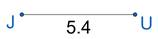Step 2 – Construct a line UM of length 4.7 cm perpendicular to the line JU.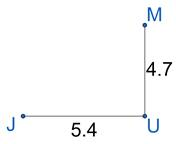Step 3 – Complete the rectangle by making JP and PM perpendicular to JU and UM respectively.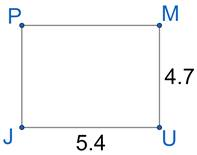Area = length × breadthA = 5.4 × 4.7A = 25.38 cm2Question 2.Construct rectangle JUMP with the following measurements. Find its area also.JU = 6 cm and JP = 5 cm.Answer:Step 1 – Construct a line JU of length 6 cm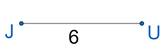Step 2 – Construct a line JP of length 5 cm perpendicular to the line JU.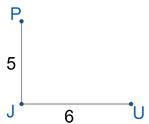Step 3 – Complete the rectangle by making UM and PM perpendicular to JU and JP respectively.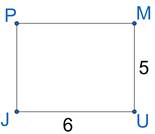Area = length × breadthA = 5 × 6A = 30 cm2Question 3.Construct rectangle JUMP with the following measurements. Find its area also.JP = 4.2 cm and MP= 2.8 cm.Answer:Step 1 – Construct a line JP of length 4.2 cm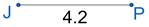Step 2 – Construct a line MP of length 2.8 cm perpendicular to the line JP.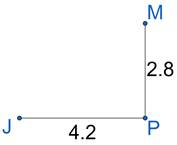Step 3 – Complete the rectangle by making JU and MU perpendicular to JP and MP respectively.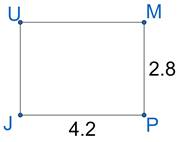Area = length × breadthA = 2.8 × 4.2A = 11.76 cm2Question 4.Construct rectangle JUMP with the following measurements. Find its area also.UM = 3.6 cm and MP = 4.6 cm.Answer:Step 1 – Construct a line MP of length 4.6 cm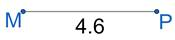Step 2 – Construct a line UM of length 3.6 cm perpendicular to the line MP.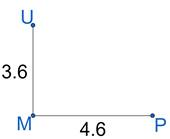Step 3 – Complete the rectangle by making JP and UJ perpendicular to MP and UM respectively.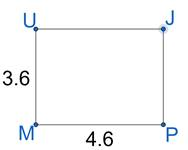Area = length × breadthA = 3.6 × 4.6A = 16.56 cm2Question 5.Construct rectangle MORE with the following measurements. Find its area also.MO = 5 cm and diagonal MR = 6.5 cm.Answer:Step 1 – Construct a line MO = 5 cm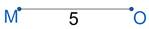Step 2 – Draw a ray perpendicular to MO passing through O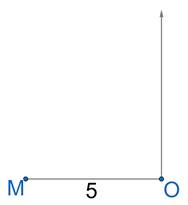Step 3 – Cut an arc of length 6.5 cm on the ray with M as center and mark the intersecting point as R.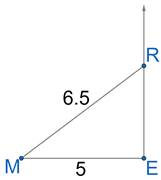Step 4 – Complete the rectangle by making RO and MO perpendicular to RE and ME respectively.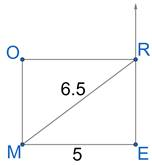Measure RE⇒ RE = 4.2 cmArea = 5 × 4.2Area = 21Question 6.Construct rectangle MORE with the following measurements. Find its area also.MO = 4.6 cm and diagonal OE = 5.4 cm.Answer:Step 1 – Construct a line MO = 4.6 cm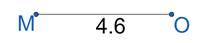Step 2 – Draw a ray perpendicular to MO passing through M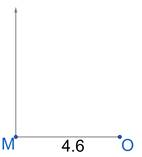Step 3 – Cut an arc of length 5.4 cm on the ray with O as center and mark the intersecting point as E.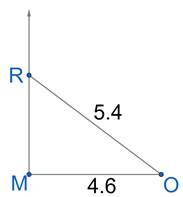Step 4 – Complete the rectangle RE and EO perpendicular to MR and MO respectively.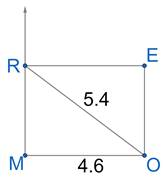Measure OE⇒ OE = 2.8 cmArea = 4.6 × 2.8Area = 12.88 cm2Question 7.Construct rectangle MORE with the following measurements. Find its area also.OR = 3 cm and diagonal MR = 5 cm.Answer:Step 1 – Construct a line OR = 3 cm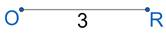Step 2 – Draw a ray perpendicular to OR passing through O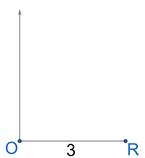Step 3 – Cut an arc of length 5 cm on the ray with R as center and mark the intersecting point as M.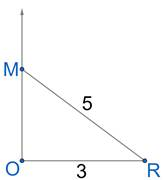Step 4 – Complete the rectangle ME and RE perpendicular to MO and OR respectively.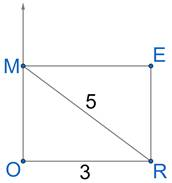Measure RE⇒ RE = 4 cmArea = 3 × 4Area = 12 cm2Question 8.Construct rectangle MORE with the following measurements. Find its area also.ME = 4 cm and diagonal OE = 6 cm.Answer:Step 1 – Construct a line OR = 3 cm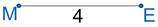Step 2 – Draw a ray perpendicular to ME passing through M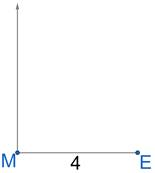Step 3 – Cut an arc of length 6 cm on the ray with E as center and mark the intersecting point as O.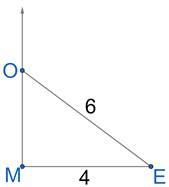Step 4 – Complete the rectangle OR and RE perpendicular to MO and ME respectively.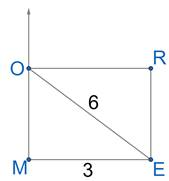Measure RE⇒ RE = 5.2 cmArea = 3 × 5.2Area = 15.6 cm2Question 9.Construct square EASY with the following measurements. Find its area also.Side 5.1 cm.Answer:Step 1 – Construct a line EA of length 5.1 cm.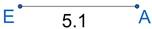Step 2 – Draw lines AS and EY perpendicular to EA of length of EA.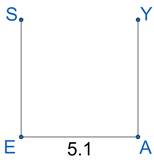Step 3 – Join S and Y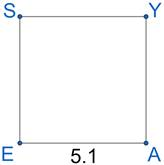Area of Square = side × side = side2Area = 5.12 = 26.01 cm2Question 10.Construct square EASY with the following measurements. Find its area also.Side 3.8 cm.Answer:Step 1 – Construct a line EA of length 3.8 cm.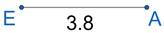Step 2 – Draw lines AS and EY perpendicular to EA of length of EA.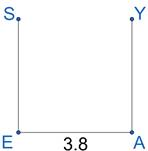Step 3 – Join S and Y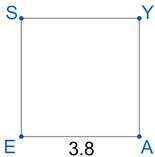Area of Square = side × side = side2Area = 3.82 = 14.44 cm2Question 11.Construct square EASY with the following measurements. Find its area also.Side 6 cm.Answer:Step 1 – Construct a line EA of length 6 cm.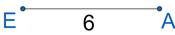Step 2 – Draw lines AS and EY perpendicular to EA of length of EA.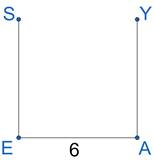Step 3 – Join S and Y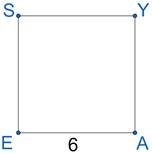Area of Square = side × side = side2Area = 62 = 36 cm2Question 12.Construct square EASY with the following measurements. Find its area also.Side 4.5 cm.Answer:Step 1 – Construct a line EA of length 4.5 cm.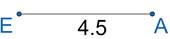Step 2 – Draw lines AS and EY perpendicular to EA of length of EA.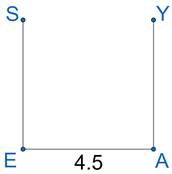Step 3 – Join S and Y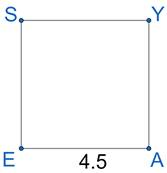Area of Square = side × side = side2Area = 4.52 = 20.25 cm2Question 13.Construct square GOLD, one of whose diagonal is given below. Find its area also.4.8 cm.Answer:Step 1 – Draw a line GL of length 4.8 cm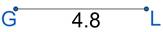Step 2 – Draw a perpendicular bisector DO of GL of same length as GL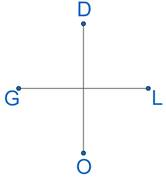HERE GL = DO = 4.8 cmStep 3 – Join the four points GOLD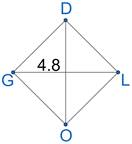Area of square =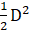(where D is the Diagonal)Area =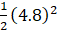Area = 11.52 cm2Question 14.Construct square GOLD, one of whose diagonal is given below. Find its area also.3.7 cm.Answer:Step 1 – Draw a line GL of length 3.7 cm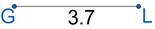Step 2 – Draw a perpendicular bisector DO of GL of same length as GL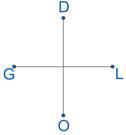HERE GL = DO = 3.7 cmStep 3 – Join the four points GOLD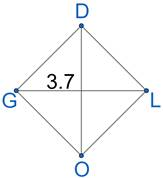Area of square =(where D is the Diagonal)Area =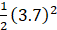Area = 6.845 cm2Question 15.Construct square GOLD, one of whose diagonal is given below. Find its area also.5 cm.Answer:Step 1 – Draw a line GL of length 5 cm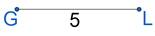Step 2 – Draw a perpendicular bisector DO of GL of same length as GL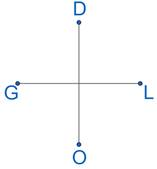HERE GL = DO = 5 cmStep 3 – Join the four points GOLD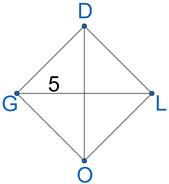Area of square =(where D is the Diagonal)Area =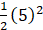Area = 12.5 cm2Question 16.Construct square GOLD, one of whose diagonal is given below. Find its area also.7 cm.Answer:Step 1 – Draw a line GL of length 7 cm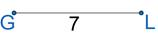Step 2 – Draw a perpendicular bisector DO of GL of same length as GL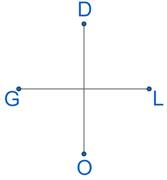HERE GL = DO = 7 cmStep 3 – Join the four points GOLD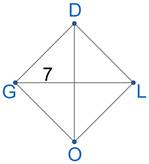Area of square =(where D is the Diagonal)Area =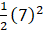Area = 24.5 cm2

PDF FILE TO YOUR EMAIL IMMEDIATELY PURCHASE NOTES & PAPER SOLUTION. @ Rs. 50/- each (GST extra)

HINDI ENTIRE PAPER SOLUTION

MARATHI PAPER SOLUTION

SSC MATHS I PAPER SOLUTION

SSC MATHS II PAPER SOLUTION

SSC SCIENCE I PAPER SOLUTION

SSC SCIENCE II PAPER SOLUTION

SSC ENGLISH PAPER SOLUTION

SSC & HSC ENGLISH WRITING SKILL

HSC ACCOUNTS NOTES

HSC OCM NOTES

HSC ECONOMICS NOTES

HSC SECRETARIAL PRACTICE NOTES

# 2019 Board Paper Solution

HSC ENGLISH SET A 2019 21st February, 2019

HSC ENGLISH SET B 2019 21st February, 2019

HSC ENGLISH SET C 2019 21st February, 2019

HSC ENGLISH SET D 2019 21st February, 2019

SECRETARIAL PRACTICE (S.P) 2019 25th February, 2019

HSC XII PHYSICS 2019 25th February, 2019

CHEMISTRY XII HSC SOLUTION 27th, February, 2019

OCM PAPER SOLUTION 2019 27th, February, 2019

HSC MATHS PAPER SOLUTION COMMERCE, 2nd March, 2019

HSC MATHS PAPER SOLUTION SCIENCE 2nd, March, 2019

SSC ENGLISH STD 10 5TH MARCH, 2019.

HSC XII ACCOUNTS 2019 6th March, 2019

HSC XII BIOLOGY 2019 6TH March, 2019

HSC XII ECONOMICS 9Th March 2019

SSC Maths I March 2019 Solution 10th Standard11th, March, 2019

SSC MATHS II MARCH 2019 SOLUTION 10TH STD.13th March, 2019

SSC SCIENCE I MARCH 2019 SOLUTION 10TH STD. 15th March, 2019.

SSC SCIENCE II MARCH 2019 SOLUTION 10TH STD. 18th March, 2019.

SSC SOCIAL SCIENCE I MARCH 2019 SOLUTION20th March, 2019

SSC SOCIAL SCIENCE II MARCH 2019 SOLUTION, 22nd March, 2019

XII CBSE - BOARD - MARCH - 2019 ENGLISH - QP + SOLUTIONS, 2nd March, 2019

# HSCMaharashtraBoardPapers2020

(Std 12th English Medium)

HSC ECONOMICS MARCH 2020

HSC OCM MARCH 2020

HSC ACCOUNTS MARCH 2020

HSC S.P. MARCH 2020

HSC ENGLISH MARCH 2020

HSC HINDI MARCH 2020

HSC MARATHI MARCH 2020

HSC MATHS MARCH 2020

# SSCMaharashtraBoardPapers2020

(Std 10th English Medium)

English MARCH 2020

HindI MARCH 2020

Hindi (Composite) MARCH 2020

Marathi MARCH 2020

Mathematics (Paper 1) MARCH 2020

Mathematics (Paper 2) MARCH 2020

Sanskrit MARCH 2020

Sanskrit (Composite) MARCH 2020

Science (Paper 1) MARCH 2020

Science (Paper 2)

Geography Model Set 1 2020-2021

MUST REMEMBER THINGS on the day of Exam

Are you prepared? for English Grammar in Board Exam.

Paper Presentation In Board Exam

How to Score Good Marks in SSC Board Exams

Tips To Score More Than 90% Marks In 12th Board Exam

How to write English exams?

How to prepare for board exam when less time is left

How to memorise what you learn for board exam

No. 1 Simple Hack, you can try out, in preparing for Board Exam

How to Study for CBSE Class 10 Board Exams Subject Wise Tips?

JEE Main 2020 Registration Process – Exam Pattern & Important Dates

NEET UG 2020 Registration Process Exam Pattern & Important Dates

How can One Prepare for two Competitive Exams at the same time?

8 Proven Tips to Handle Anxiety before Exams!